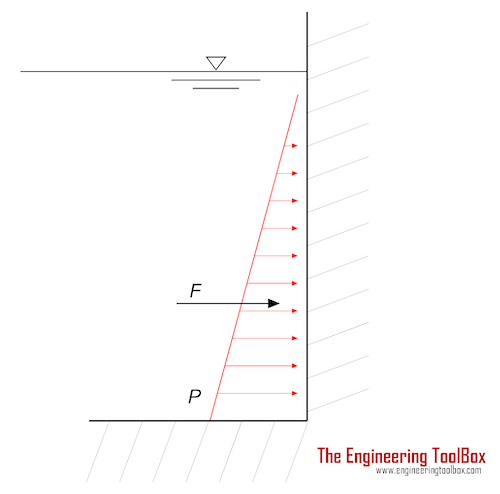Engineering ToolBox - Resources, Tools and Basic Information for Engineering and Design of Technical Applications!

# Hydrostatic Force acting on Submerged Surface

## Calculate the thrust force acting on a submerged surface.The thrust force acting on a surface submerged in a liquid can be calculated as

F = pa

=ρ g ha A                           (1)

where

F = thrust force (N)

pa = ρ g ha = average pressure on the surface (Pa)

A = area of submerged surface (m2)

ha = average depth (m)

ρ = density (kg/m3)  (water 1000 kg/m3)

g = acceleration of gravity (9.81 m/s2

### Example - The thrust force acting on the side of a container

The trust force acting on the submerged vertical side of a container can be calculated as

F = pa

= ((pt + pb) / 2) A

= (ρ g (ht + hb) / 2) A                         (2)

where

pt = pressure at the top of the submerged surface (Pa)

pb = pressure at the bottom of the submerged surface (Pa)

ht = depth at the top of the submerged surface (m)

hb = depth at the bottom of the submerged surface (m)

The thrust on a surface with width 1 m and height from 0 m to 2 m - in a water-filled container can be calculated as

F =  (ρ g (ht + hb) / 2) A

= (1000 kg/m3) (9.81 m/s2) ((0 m) + (2 m)) / 2) ((1 m) (2 m))

= 19620 N

= 19.6 kN

### Example - The thrust force acting on the bottom of a container

The trust force acting on the horizontal bottom of a submerged container can be calculated as

F =  pb A

= ρ g hb A                                     (2b)

The thrust on a bottom with width 1 m and length 2 m - on depth 1 m - in a water filled container can be calculated as

F =  ρ g hb A

= (1000 kg/m3) (9.81 m/s2) (1 m) ((1 m) (2 m))

= 19620 N

= 19.6 kN

## Related Topics

• ### Fluid Mechanics

The study of fluids - liquids and gases. Involving velocity, pressure, density and temperature as functions of space and time.

## Related Documents

• ### Archimedes' Law

Forces acting on bodies submerged in fluids.
• ### Buoyancy

Buoyancy is the resultant force acting on a submerged body.
• ### Center of Gravity

A body and the center of gravity.
• ### Center of Gravity and Buoyancy

Stability - the center of gravity vs. the center of buoyancy.
• ### Hydrostatic Pressure vs. Depth

Depth and hydrostatic pressure.
• ### Underground Pipes - Earth Pressure

Pressure acting on underground pipes due to weight of soil and transport.

## Engineering ToolBox - SketchUp Extension - Online 3D modeling!

Add standard and customized parametric components - like flange beams, lumbers, piping, stairs and more - to your Sketchup model with the Engineering ToolBox - SketchUp Extension - enabled for use with older versions of the amazing SketchUp Make and the newer "up to date" SketchUp Pro . Add the Engineering ToolBox extension to your SketchUp Make/Pro from the Extension Warehouse !

We don't collect information from our users. More about

## Citation

• The Engineering ToolBox (2011). Hydrostatic Force acting on Submerged Surface. [online] Available at: https://www.engineeringtoolbox.com/thrust-submerged-surface-d_1767.html [Accessed Day Month Year].

Modify the access date according your visit.

9.29.12